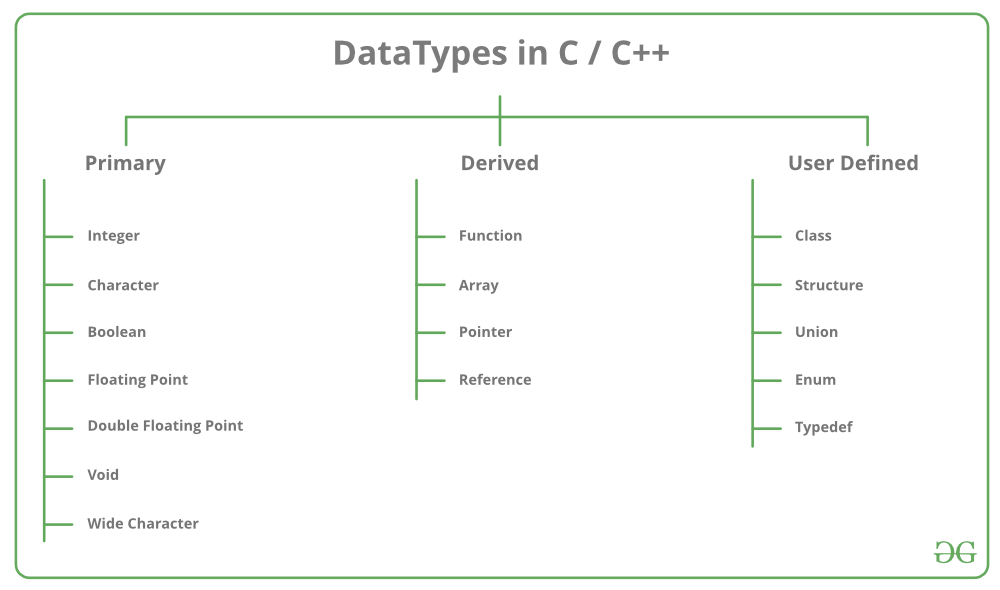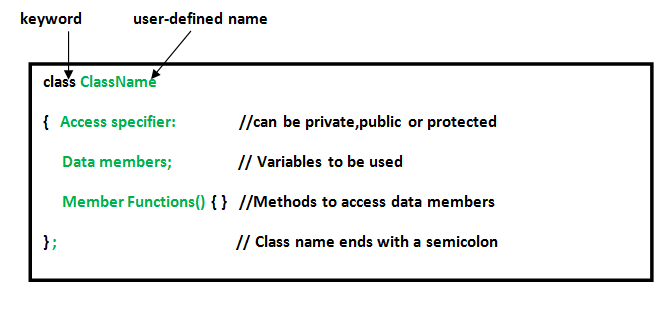Open in App
Not now

# User defined Data Types in C++

• Difficulty Level : Easy
• Last Updated : 28 Jun, 2021

Data types are means to identify the type of data and associated operations of handling it. There are three types of data types:

1. Pre-defined DataTypes
2. Derived Data Types
3. User-defined DataTypes### User-Defined DataTypes:

The data types that are defined by the user are called the derived datatype or user-defined derived data type.
These types include:

Below is the detailed description of the following types:

1. Class: The building block of C++ that leads to Object-Oriented programming is a Class. It is a user-defined data type, which holds its own data members and member functions, which can be accessed and used by creating an instance of that class. A class is like a blueprint for an object.

Syntax:Example:

 `// C++ program to demonstrate ` `// Class ` ` `  `#include ` `using` `namespace` `std; ` ` `  `class` `Geeks { ` `    ``// Access specifier ` `public``: ` `    ``// Data Members ` `    ``string geekname; ` ` `  `    ``// Member Functions() ` `    ``void` `printname() ` `    ``{ ` `        ``cout << ``"Geekname is: "` `<< geekname; ` `    ``} ` `}; ` ` `  `int` `main() ` `{ ` ` `  `    ``// Declare an object of class geeks ` `    ``Geeks obj1; ` ` `  `    ``// accessing data member ` `    ``obj1.geekname = ``"GeeksForGeeks"``; ` ` `  `    ``// accessing member function ` `    ``obj1.printname(); ` ` `  `    ``return` `0; ` `} `

Output:

```Geekname is: GeeksForGeeks
```
2. Structure: A structure is a user defined data type in C/C++. A structure creates a data type that can be used to group items of possibly different types into a single type.

Syntax:

```struct address {
char name;
char street;
char city;
char state;
int pin;
};```

Example:

 `// C++ program to demonstrate ` `// Structures in C++ ` ` `  `#include ` `using` `namespace` `std; ` ` `  `struct` `Point { ` `    ``int` `x, y; ` `}; ` ` `  `int` `main() ` `{ ` `    ``// Create an array of structures ` `    ``struct` `Point arr; ` ` `  `    ``// Access array members ` `    ``arr.x = 10; ` `    ``arr.y = 20; ` ` `  `    ``cout << arr.x << ``", "` `<< arr.y; ` ` `  `    ``return` `0; ` `} `

Output:

```10, 20
```
3. Union: Like Structures, union is a user defined data type. In union, all members share the same memory location. For example in the following C program, both x and y share the same location. If we change x, we can see the changes being reflected in y.

 `#include ` `using` `namespace` `std; ` ` `  `// Declaration of union is same as the structures ` `union` `test { ` `    ``int` `x, y; ` `}; ` ` `  `int` `main() ` `{ ` `    ``// A union variable t ` `    ``union` `test t; ` ` `  `    ``// t.y also gets value 2 ` `    ``t.x = 2; ` ` `  `    ``cout << ``"After making x = 2:"` `         ``<< endl ` `         ``<< ``"x = "` `<< t.x ` `         ``<< ``", y = "` `<< t.y ` `         ``<< endl; ` ` `  `    ``// t.x is also updated to 10 ` `    ``t.y = 10; ` ` `  `    ``cout << ``"After making Y = 10:"` `         ``<< endl ` `         ``<< ``"x = "` `<< t.x ` `         ``<< ``", y = "` `<< t.y ` `         ``<< endl; ` ` `  `    ``return` `0; ` `} `

Output:

```After making x = 2:
x = 2, y = 2
After making Y = 10:
x = 10, y = 10
```
4. Enumeration: Enumeration (or enum) is a user defined data type in C. It is mainly used to assign names to integral constants, the names make a program easy to read and maintain.

Syntax:

`enum State {Working = 1, Failed = 0}; `

 `// Program to demonstrate working ` `// of enum in C++ ` ` `  `#include ` `using` `namespace` `std; ` ` `  `enum` `week { Mon, ` `            ``Tue, ` `            ``Wed, ` `            ``Thur, ` `            ``Fri, ` `            ``Sat, ` `            ``Sun }; ` ` `  `int` `main() ` `{ ` `    ``enum` `week day; ` ` `  `    ``day = Wed; ` ` `  `    ``cout << day; ` ` `  `    ``return` `0; ` `} `

Output:

```2
```
5. Typedef : C++ allows you to define explicitly new data type names by using the keyword typedef. Using typedef does not actually create a new data class, rather it defines a name for an existing type. This can increase the portability(the ability of a program to be used across different types of machines; i.e., mini, mainframe, micro, etc; without much changes into the code)of a program as only the typedef statements would have to be changed. Using typedef one can also aid in self-documenting code by allowing descriptive names for the standard data types.

Syntax:

`typedef type name;`

where type is any C++ data type and name is the new name for this data type.
This defines another name for the standard type of C++.

Example:

 `// C++ program to demonstrate typedef ` `#include ` `using` `namespace` `std; ` ` `  `// After this line BYTE can be used ` `// in place of unsigned char ` `typedef` `unsigned ``char` `BYTE``; ` ` `  `int` `main() ` `{ ` `    ``BYTE` `b1, b2; ` `    ``b1 = ``'c'``; ` `    ``cout << ``" "` `<< b1; ` `    ``return` `0; ` `} `

Output:

```c
```

6. My Personal Notes arrow_drop_up
Related Articles